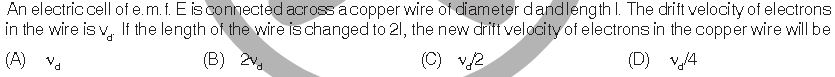# WBJEE '13 Q.•Case 1)L=12aT2
and Vd=L/T

Case 2) 2L=12at2

time for accn of e is diff in the 2 cases, so Vd will be affected

and using the formula i=neA(Vd) , where A is area and equating volume, new Vd can be obtained

••